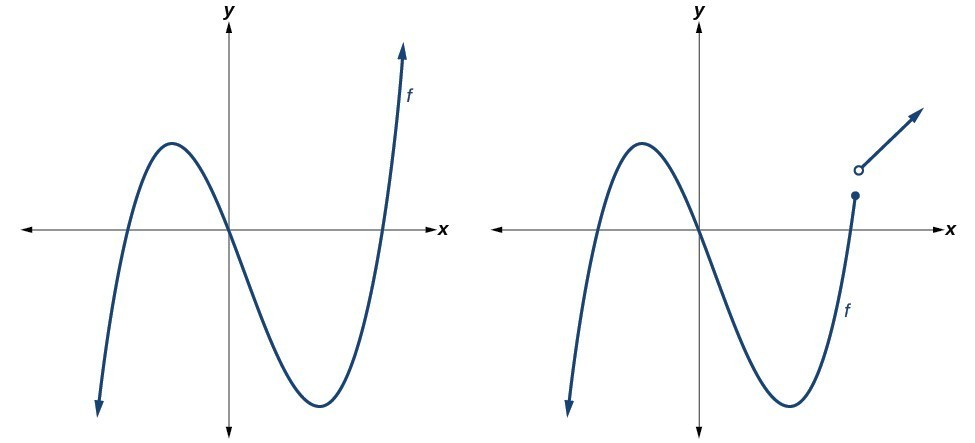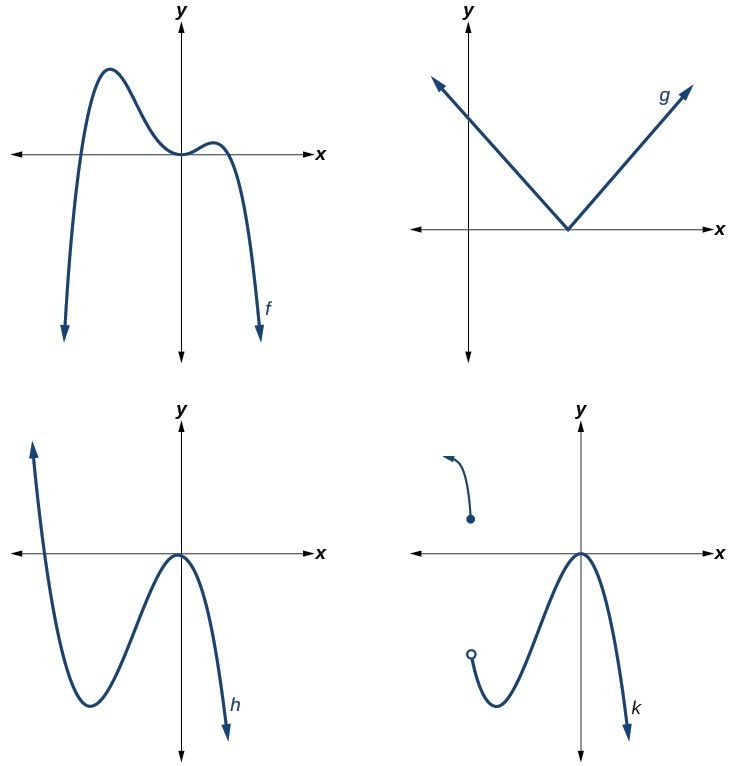## Recognize characteristics of graphs of polynomial functions

Polynomial functions of degree 2 or more have graphs that do not have sharp corners; recall that these types of graphs are called smooth curves. Polynomial functions also display graphs that have no breaks. Curves with no breaks are called continuous. Figure 1 shows a graph that represents a polynomial function and a graph that represents a function that is not a polynomial.Figure 1

### Example 1: Recognizing Polynomial Functions

Which of the graphs in Figure 2 represents a polynomial function?Figure 2

### Solution

The graphs of f and h are graphs of polynomial functions. They are smooth and continuous.

The graphs of g and are graphs of functions that are not polynomials. The graph of function g has a sharp corner. The graph of function k is not continuous.

### Q & A

Do all polynomial functions have as their domain all real numbers?

Yes. Any real number is a valid input for a polynomial function.